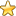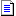The Main Number Sections:
0 - 500 | 501 - 1000 | 1001 - 1500 | 1501 - 2000 | 2001 - 2500
Number Articles | Higher Numbers | Random Number
You are here: HomeThe Database of Number Correlations# Prime Numbers And The Fundamental Theorem of Arithmetic

by Joe Pagano

Just as atoms are the building blocks of elements, prime numbers are the building blocks of arithmetic. This comparison becomes justified when we consider that all composite numbers can be uniquely generated by a specific product of primes. This last statement is one of the most important in number theory and is known as the Fundamental Theorem of Arithmetic.

If you have forgotten your definitions, a prime number is any number which is divisible by only the number 1 and itself. The first few primes are 2, 3, 5, 7, 11, and 13; 2 being the only even prime. A composite number is a number that has at least one other factor besides the number 1 and itself. It should be noted that in order for a number to be prime it must be odd, but not all odd numbers are primes. The Fundamental Theorem of Arithmetic states that every composite number can be written uniquely-without regard to order of the factors-by a product of prime numbers.

Take the number 56 for example. We can break this down using a factor tree and come up with the following prime number decomposition: 2 x 2 x 2 x 7. When breaking a composite number down into prime factors, all you really need do is familiarize yourself with the first 10 primes or so. These are 2, 3, 5, 7, 11, 13, 17, 19, 23, 29. If a number is even, such as 56, keep breaking it down by 2's until you cannot; then proceed to eliminate the other prime factors accordingly. To make this procedure clearer, take the composite number 212. Since it is even, break it into
2 x 106. Since 106 is even, break this into 2 x 53. Since 53 is prime, we write
212 = 2 x 2 x 53.

Historically, primes were considered of interest for purely mathematical reasons. It was not until the 1970's when prime number theory found itself intricately entwined with RSA Cryptography, or the encoding of data. Now primes are recognized as integral to the internet commerce infrastructure by allowing secure transactions via computers. Without a solid understanding of these curious mathematical numbers, secure e-commerce would not be possible.

The Fundamental Theorem of Arithmetic also provides us with an algorithm for finding the least common denominator, LCD, of two numbers, which is used when adding or subtracting fractions. For example, suppose we have 15 and 39. We can decompose these numbers into primes as follows: 15 = 3 x 5 and 39 = 3 x 13. We can find the LCD by taking the product of the distinct primes and only using the common primes once. Thus since 3, 5, and 13 are distinct, and 3 is used twice, we find the LCD by writing the product of these numbers to get LCD = 3 x 5 x 13 = 195.

So the next time you think a number is a number is a number. Remember this is not the case at all. The wonderful world of primes teaches us that no type of number should be taken for granted. For who knows? Maybe if you give it enough thought, you might be able to find some exotic application for some types of numbers that are just studied now for purely mathematical reasons. Then you could become famous too.

See more of my articles here at Articles Page
and see my challenging brainteasers here at Problem of the Week and Cool Brain Teasers
 Joe is a prolific writer of self-help and educational material and an award-winning former teacher of both college and high school mathematics. Joe is the creator of the Wiz Kid series of math ebooks, Arithmetic Magic, the little classic on the ABC's of arithmetic, the original collection of poetry, Poems for the Mathematically Insecure, and the short but highly effective fraction troubleshooter Fractions for the Faint of Heart. The diverse genre of his writings (novel, short story, essay, script, and poetry)-particularly in regard to its educational flavor- continues to captivate readers and to earn him recognition.Joe propagates his teaching philosophy through his articles and books and is dedicated to helping educate children living in impoverished countries. Toward this end, he donates a portion of the proceeds from the sale of every ebook. For more information go to http://www.mathbyjoe.com

You are here: HomeThe Database of Number CorrelationsThe Main Number Sections:
0 - 500 | 501 - 1000 | 1001 - 1500 | 1501 - 2000 | 2001 - 2500
Number Articles | Higher Numbers | Random NumberSearch on Amazon:
prime numbers or...?